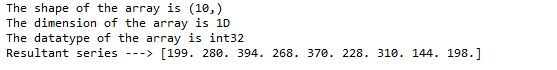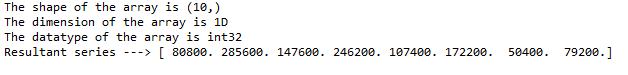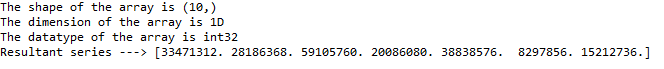# Differentiate a Chebyshev series using NumPy in Python

• Difficulty Level : Expert
• Last Updated : 06 May, 2022

In this article, we will cover how to integrate a Chebyshev series and set the integration constant in Python using NumPy.

## chebyshev.chebder method

The Chebyshev series has polynomials with the largest possible leading coefficient, whose absolute value on the interval [−1, 1] is bounded by 1. They are also the “extremal” polynomials. Chebyshev polynomials are significant in approximation theory because the roots of Tn(x), which are also called Chebyshev nodes, are used as matching points for optimizing polynomial interpolation. The resulting interpolation polynomial minimizes the problem of Runge’s phenomenon and provides an approximation that is close to the best polynomial approximation to a continuous function under the maximum norm, also called the “minimax” criterion.

In python, to perform Chebyshev differentiation, NumPy provides a function called chebyshev.chebder which can be used to integrate the Chebyshev series. This function returns the Chebyshev series coefficients c differentiated m times along the axis.

Syntax: chebyshev.chebder(c, m=1, scl=1, axis=0)

Parameters:

• c – an array of Chebyshev series coefficients
• m –  no of derivatives taken, must be non-negative
• scl – scaler (linear) value, Each differentiation is multiplied by scl.
• axis – Axis over which the derivative is taken. default – 0

Return: Chebyshev series

### Example 1:

In the first example. let us consider a 1D array with a first-order derivative and 1 as a scaling constant. Import the necessary packages as shown and pass the appropriate parameters as shown below.

## Python3

 `import` `numpy as np` `from` `numpy.polynomial ``import` `chebyshev`   `# co.efficient array` `c ``=` `np.array([``1``, ``2``, ``3``, ``4``, ``5``, ``6``, ``7``, ``8``, ``9``, ``11``])`   `print``(f``'The shape of the array is {c.shape}'``)` `print``(f``'The dimension of the array is {c.ndim}D'``)` `print``(f``'The datatype of the array is {c.dtype}'``)`   `res ``=` `chebyshev.chebder(c, m``=``1``, scl``=``1``)`   `# differentiated chebyshev series with ` `# first order derivative and scale 1` `print``(f``'Resultant series ---> {res}'``)`

Output:Output

### Example 2:

In the second example. let us consider a 1D array with a second-order derivative and 5 as a scaling constant. Import the necessary packages as shown and pass the appropriate parameters as shown below.

## Python3

 `import` `numpy as np` `from` `numpy.polynomial ``import` `chebyshev`   `# co.efficient array` `c ``=` `np.array([``1``, ``2``, ``3``, ``4``, ``5``, ``6``, ``7``, ``8``, ``9``, ``11``])`   `print``(f``'The shape of the array is {c.shape}'``)` `print``(f``'The dimension of the array is {c.ndim}D'``)` `print``(f``'The datatype of the array is {c.dtype}'``)`   `res ``=` `chebyshev.chebder(c, m``=``2``, scl``=``5``)`   `# differentiated chebyshev series with` `# second order derivative and scale 5` `print``(f``'Resultant series ---> {res}'``)`

Output:Output

### Example 3:

In the third example. let us consider a 1D array with a third-order derivative and 7 as a scaling constant. Import the necessary packages as shown and pass the appropriate parameters as shown below.

## Python3

 `import` `numpy as np` `from` `numpy.polynomial ``import` `chebyshev`   `# co.efficient array` `c ``=` `np.array([``1``, ``2``, ``3``, ``4``, ``5``, ``6``, ``7``, ``8``, ``9``, ``11``])`   `print``(f``'The shape of the array is {c.shape}'``)` `print``(f``'The dimension of the array is {c.ndim}D'``)` `print``(f``'The datatype of the array is {c.dtype}'``)`   `res ``=` `chebyshev.chebder(c, m``=``3``, scl``=``7``)`   `# differentiated chebyshev series with` `# third  order derivative and scale 7` `print``(f``'Resultant series ---> {res}'``)`

Output:Output

My Personal Notes arrow_drop_up
Recommended Articles
Page :